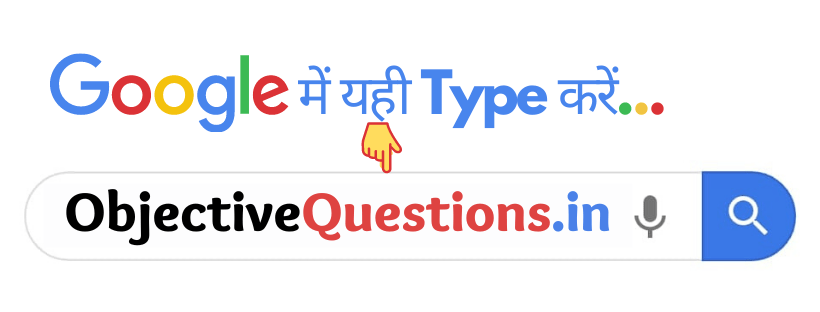# Input Output Statements

1. What is an alternative name for an object?

• Function
• Variable
• Reference
• Initialization
Reference

2. The Const modifier on its own is equivalent to :

• Const float
• Const int
• Const Char
• None of these
Const int

3. Setw ( ) and Setprecision ( ) manipulators are use to ?

• Formate output data
• Formate Processing data
•  Formate Input data
•  None of the these
Formate output data

4. Which data type is used for an empty set of values and non – returning function?

• Void data types
• float data types
•  Double data types
• None of these
Void data types

5. Which of the following has no reference?

• Array
• Pointer
• Reference
•  All of these
All of these

6. A memory location that is shared by two or more different variables is known as :

• Enumeration
• Union
• Structure
• Class
Union

7. To identify the type of data and associated operations of handling it is known as :

• Data function
• Data type
•  Data handing
• None of these
Data type

8. Which of the following is not a user-defined derived data type?

• Union
•  Structure
•  Class
• Function
Function

9. Binary search algorithm uses :

• Divide and conquer method
•  Bubble sorting technique
• Linear way to search values
• None of these
Divide and conquer method

10. Sequence of objects that have same type is called :

•  Stack
• Array
• Operator
• Function
Array

11. Which is a type of data type?

• Derived types
•  Fundamental type
• Both a and b
• None of these
Both a and b

12. Who describes all the properties of a data type?

• Structure
• Main
• Object
• Class
Class

13. An alternative method for naming integer constants is known as : Computer Sc

• Enumeratien
•  Union
• Function
• Structure
Enumeratien

14. ASCII value of Ais :

• 64
• 65
• 96
•  97
65

15. What is the size of the w chart in C ++?

• 4
• 2 or 4
•  2
• Based on the number of bits in the system
Based on the number of bits in the system

16. The data type that is not composed of other data types is known as :

•  Derived data types
•  Composed data types
• Fundamental data types
• None of the above
Fundamental data types

17. The minimum range of unsigned char is :

•  0 to 255
•  0 to 244
•  -128 to 127
• none of these